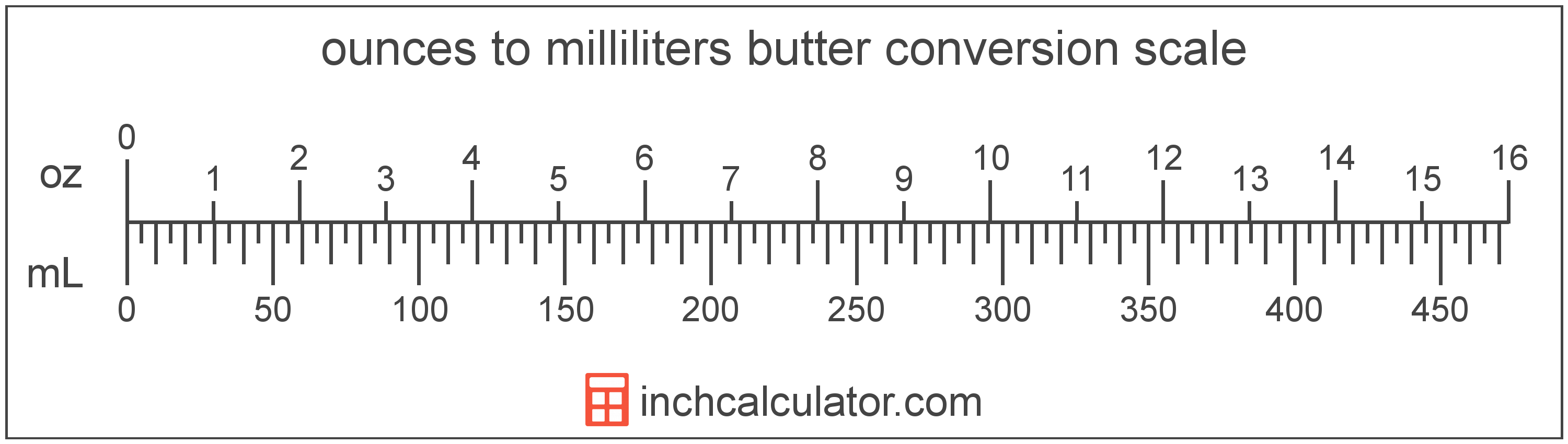# Convert Ounces of Butter to Milliliters

Enter the butter in ounces below to get the value converted to milliliters.

Results in Milliliters:1 oz = 29.57353 mL

Do you want to convert milliliters to ounces?

## How to Convert Ounces to Milliliters

To convert a measurement in ounces to a measurement in milliliters, multiply the butter by the following conversion ratio: 29.57353 milliliters/ounce.

Since one ounce of butter is equal to 29.57353 milliliters, you can use this simple formula to convert:

milliliters = ounces × 29.57353

The butter in milliliters is equal to the butter in ounces multiplied by 29.57353.

For example, here's how to convert 5 ounces to milliliters using the formula above.
milliliters = (5 oz × 29.57353) = 147.86765 mL### How Many Milliliters Are in an Ounce of Butter?

There are 29.57353 milliliters in an ounce of butter, which is why we use this value in the formula above.

1 oz = 29.57353 mL

## What Is an Ounce?

In the US butter is sold in 16 ounce packages, which contain 1 pound of butter.

The ounce is a US customary and imperial unit of weight. Ounces can be abbreviated as oz; for example, 1 ounce can be written as 1 oz.

## What Is a Milliliter?

Butter is sometimes measured by volume in milliliters. One stick of butter contains about 118.3 milliliters.

The milliliter is an SI unit of volume in the metric system. A milliliter is sometimes also referred to as a millilitre. Milliliters can be abbreviated as mL; for example, 1 milliliter can be written as 1 mL.

## Ounce to Milliliter Conversion Table

Table showing various ounce measurements converted to milliliters.
Ounces Milliliters
1 oz 29.57 mL
2 oz 59.15 mL
3 oz 88.72 mL
4 oz 118.29 mL
5 oz 147.87 mL
6 oz 177.44 mL
7 oz 207.01 mL
8 oz 236.59 mL
9 oz 266.16 mL
10 oz 295.74 mL
11 oz 325.31 mL
12 oz 354.88 mL
13 oz 384.46 mL
14 oz 414.03 mL
15 oz 443.6 mL
16 oz 473.18 mL
17 oz 502.75 mL
18 oz 532.32 mL
19 oz 561.9 mL
20 oz 591.47 mL
21 oz 621.04 mL
22 oz 650.62 mL
23 oz 680.19 mL
24 oz 709.76 mL
25 oz 739.34 mL
26 oz 768.91 mL
27 oz 798.49 mL
28 oz 828.06 mL
29 oz 857.63 mL
30 oz 887.21 mL
31 oz 916.78 mL
32 oz 946.35 mL
33 oz 975.93 mL
34 oz 1,006 mL
35 oz 1,035 mL
36 oz 1,065 mL
37 oz 1,094 mL
38 oz 1,124 mL
39 oz 1,153 mL
40 oz 1,183 mL